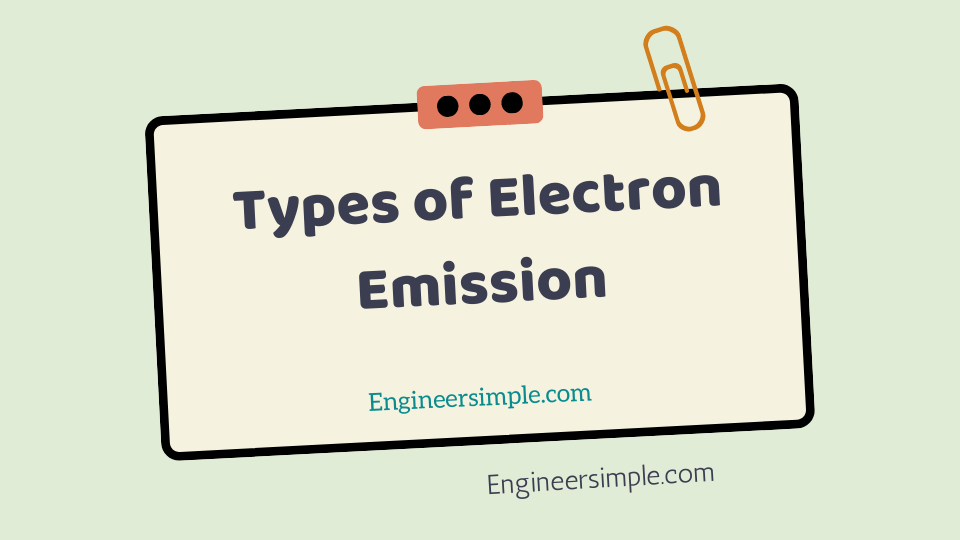#The electron emission from the surface of a metal is possible only if sufficient additional
energy (equal to the work function of the metal) is supply from some external source.
This external energy may come from a variety of sources such as heat energy, energy
stored in electric field, light energy or kinetic energy of the electric charges bombarding
the metal surface. Accordingly, there are following our principal methods of obtaining
electron emission from the surface of a metal:
…………………………………………………………………………………………………………………

### Work function is the additional energy require for the liberation of electrons.

Types of Electron Emission .Therefore, it should have the conventional unit of energy le. Joules. But this unit
is very large for computing electronics work. Therefore, in practice, a smaller unit
called electron volt (abbreviated as eV) is use. One electron-volt is the amount

of energy acquired by an electron when it is accelerate through a potential
difference of 1 V.

Thus, if an electron moves from a point of potential to a point of +10V, then amount of
energy acquired by the electron is 10 eV.
Since charge on an electron = 1.602 x 10 -19 C and voltage = 1 V,
1 electron-volt = Q V = (1.602 x 10 -19 ) x 1 J

##### or 1 eV = 1.602 x 10 -19 J

(i) Thermionic emission. In this method, the metal is heate to sufficient
temperature (about 2500 0 C) to enable the free electrons to leave the metal surface. The
number of electrons emitted depends upon the temperature. The higher the
temperature, the greater is the emission of electrons. This type of emission is employed
in vacuum tubes.

(ii) Field emission. In this method, a strong electric field (i.e. a high positive
voltage) is applied at the metal surface which pulls the free electrons out of metal
because of the attraction of positive field. The stronger the electric field, the greater is
the electron emission.

(iii) Photo-electric emission. In this method, the energy of light falling upon the
metal surface is transferred to the free electrons within the metal to enable them to
leave the surface. The greater the intensity (i.e. brightness) of light beam falling on the
metal surface, the greater the photo-electric emission.

(iv) Secondary emission. In this method, a high velocity beam of electrons
strikes the metal surface and causes the free electrons of the metal to be knocked out
from the surface.